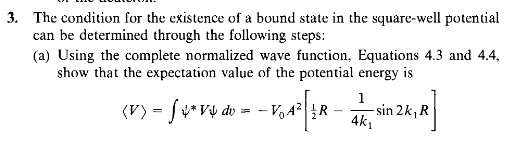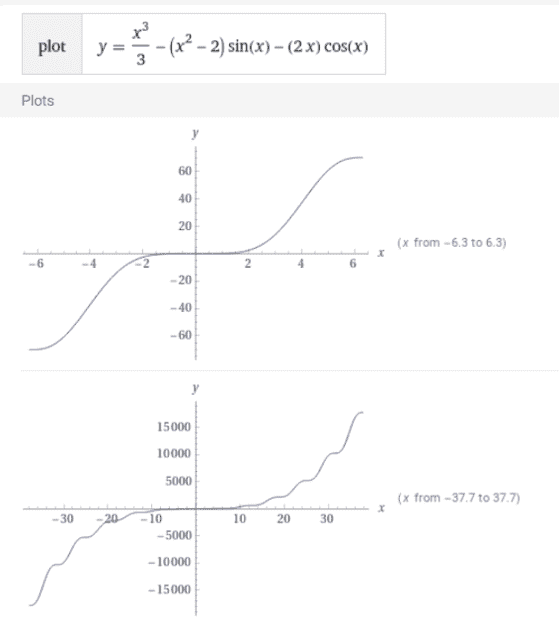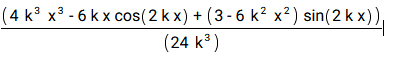# Expectation of Kinetic Energy for Deuteron

James_1978
Homework Statement:
I am solving for the expectation energy for the deuteron. However, I must be missing something because my answer is very complicated compared to what is expected. I am looking at problem 4.3 from Krane.
Relevant Equations:
## <T> = \frac{\hbar^{2}}{2m} \int_{0}^{\infty} |\frac{\partial\psi}{\partial r}|^{2} ##
## <T> = \frac{\hbar^{2}}{2m} A^{2} [\frac{1}{2} k_{1}^{2} + \frac{1}{4}\sin{2k_{1}r} + \frac{k_{2}}{2}\sin{k_{1}r}^{2} ]##
##A\sin{k_{1}r}## for ##r < R##
##Ce^{-k_{2}r}## for ##r > R##
Dear Forum,

I am solving for the expectation value of the kinetic energy for the deuteron (Krane problem 4.3). I must be missing something since this has become far more complicated than I remember.

The problem is as follows:

## <T> = \frac{\hbar^{2}}{2m} \int_{0}^{\infty} |\frac{\partial\psi}{\partial r}|^{2} ##

And show

## <T> = \frac{\hbar^{2}}{2m} A^{2} [\frac{1}{2} k_{1}^{2} + \frac{1}{4}\sin{2k_{1}r} + \frac{k_{2}}{2}\sin{k_{1}r}^{2} ]##

The correct way (I believe) is to use the Laplacian with the wave functions for the deuteron which are:

##A\sin{k_{1}r}## for ##r < R##

And

##Ce^{-k_{2}r}## for ##r > R##

My attemp is as follows. For the kinetic energy we have ##<T> = <\frac{p^{2}}{2m}>## Where ##p = i\hbar\nabla^{2}## To solve for the expectation value I do the following.

##<T> = \int\Psi^{*}\nabla^{2}\Psi d^{3}\overrightarrow{r}##

##<T> = \int\Psi^{*}\frac{1}{r^{2}}\frac{\partial}{\partial r}(r^{2}\frac{\partial}{\partial r})\Psi d^{3}\overrightarrow{r}##

##<T> =\frac{-\hbar^{2}}{2m}[ \int_{0}^{R}A^{*}\sin{k_{1}}\frac{1}{r^{2}}\frac{\partial}{\partial r}(r^{2}\frac{\partial}{\partial r})A\sin{k_{1}}r^{2}dr + \int_{R}^{\infty}C^{*}e^{-k_{2}r}\frac{1}{r^{2}}\frac{\partial}{\partial r}(r^{2}\frac{\partial}{\partial r})Ce^{-k_{2}r}r^{2}dr]##

I understand we don't need to include the Angular components since they vannish with a symmetric wave function. What I don't understand is that when I do this above the equations becomes very complicated and does not reduce down as expected. However, I think we are only asked to do the following.

## <T> = \frac{\hbar^{2}}{2m} \int_{0}^{\infty} |\frac{\partial\psi}{\partial r}|^{2} = \frac{-\hbar^{2}}{2m}[ \int_{0}^{R}A^{2}\sin^{2}{k_{1}}dr + \int_{R}^{\infty}C^{2}e^{-2k_{2}r}dr]##

My question why are we not supposed to apply the Laplacian? The answers works out but I am not sure why I do not need to use the Laplacian.

Gold Member
I expect they coincide making use of partial integration. Have you invesigated it ?

James_1978
Dear Anuttarasammyak, I am not sure what you mean by partial investigation. Why when you square the radial momentum operator does the ##1/r## and ##r## not squared as well?

Gold Member
$$\frac{\hbar^{2}}{2m} \int_{0}^{\infty} 4\pi r^2dr |\frac{\partial\psi}{\partial r}|^{2}$$
$$= \frac{\hbar^{2}}{2m} \int_{0}^{\infty} 4\pi r^2dr \frac{\partial\psi^*}{\partial r} \frac{\partial\psi}{\partial r}$$
$$= \frac{\hbar^{2}}{2m} [4\pi r^2 \psi^* \frac{\partial\psi}{\partial r}]_0^\infty-\frac{\hbar^{2}}{2m} \int_{0}^{\infty} dr \psi^* \frac{\partial}{\partial r} (4\pi r^2 \frac{\partial \psi}{\partial r}) =-\frac{\hbar^{2}}{2m} \int_{0}^{\infty} dr \psi^* \frac{\partial}{\partial r} (4\pi r^2 \frac{\partial \psi}{\partial r})$$
Won't you investigate whether it coincides with applying Laplacian ?

Last edited:
James_1978
Thank you. However, I am still a little confused. Your last post makes much more sense. But, this and the Laplacian are different. Am I still missing something?

Gold Member
Isn't is same with your
$$<T> = \int\Psi^{*}\frac{1}{r^{2}}\frac{\partial}{\partial r}(r^{2}\frac{\partial}{\partial r})\Psi d^{3}\overrightarrow{r}$$ multiplied by ##-\hbar^2/2m## ?

James_1978
Yes. You are saying that this is the same as what you have in post #4? That is integration by parts. By the way in post #4 your middle term and the last term in the last equation are the same. Is that correct?

•anuttarasammyak
Gold Member
It said ##[\ \ \ ]_0^\infty## term is zero. I hope you have cleared the question.

James_1978
Yes, that is right. But the last term in the last equation in post #4 is what we are supposed to use. I am still not connecting that to the Laplacian. Are you saying

##<T> = \int\psi^{*}\frac{1}{r^{2}}\frac{\partial}{\partial r}(r^{2}\frac{\partial}{\partial r})\psi d^{3}\overrightarrow{r}##

is equal to

##<T> = \int\psi^{*}\frac{\partial^{2}\psi}{\partial r^{2}} d^{3}\overrightarrow{r}##

Gold Member
$$\frac{\hbar^{2}}{2m} \int_{0}^{\infty} 4\pi r^2dr |\frac{\partial\psi}{\partial r}|^{2}$$
$$= \frac{\hbar^{2}}{2m} \int_{0}^{\infty} 4\pi r^2dr \frac{\partial\psi^*}{\partial r} \frac{\partial\psi}{\partial r}$$
$$= \frac{\hbar^{2}}{2m} [4\pi r^2 \psi^* \frac{\partial\psi}{\partial r}]_0^\infty-\frac{\hbar^{2}}{2m} \int_{0}^{\infty} dr \psi^* \frac{\partial}{\partial r} (4\pi r^2 \frac{\partial \psi}{\partial r}) =-\frac{\hbar^{2}}{2m} \int_{0}^{\infty} dr \psi^* \frac{\partial}{\partial r} (4\pi r^2 \frac{\partial \psi}{\partial r})$$
Won't you investigate whether it coincides with applying Laplacian ?
It starts from the form that the text applies and ends with applying Laplacian which you described in OP, i.e. $$<T> = \int\Psi^{*}\frac{1}{r^{2}}\frac{\partial}{\partial r}(r^{2}\frac{\partial}{\partial r})\Psi d^{3}\overrightarrow{r}$$ multiplied by ##-\hbar^2/2m##

Last edited:
James_1978
I see what you are saying now. I will try that. I guess I also got confused when I put the ##r^{2}## after the operator.

•anuttarasammyak
James_1978
I have a question. In post #2 you square the radial momentum operator. Why is the ##1/r## and ##r## not squared?

Gold Member
$$\frac{\hbar^{2}}{2m} \int_{0}^{\infty} 4\pi r^2dr |\frac{\partial\psi}{\partial r}|^{2}$$
$$= \frac{\hbar^{2}}{2m} \int_{0}^{\infty} 4\pi r^2dr \frac{\partial\psi^*}{\partial r} \frac{\partial\psi}{\partial r}$$
$$= \frac{\hbar^{2}}{2m} [4\pi r^2 \psi^* \frac{\partial\psi}{\partial r}]_0^\infty-\frac{\hbar^{2}}{2m} \int_{0}^{\infty} dr \psi^* \frac{\partial}{\partial r} (4\pi r^2 \frac{\partial \psi}{\partial r}) =-\frac{\hbar^{2}}{2m} \int_{0}^{\infty} dr \psi^* \frac{\partial}{\partial r} (4\pi r^2 \frac{\partial \psi}{\partial r})$$
Won't you investigate whether it coincides with applying Laplacian ?
That’s all the formula I wrote. Which one are you referring ?

James_1978
This one

##-\hbar^2 \frac{1}{r}\frac{\partial^2 }{\partial r^2}r##

Gold Member
....Now I cannot find it in my lines.

James_1978
you wrote it yesterday.

Gold Member
If so please disregard it. Now I do not need that formula. I apologize my bad.

[EDIT]
Some texts refer to "radial momentum operator" which is Hermitian and becomes radial component of Laplacian when squared and divided by 2m.
$$\frac{\hbar}{i} \frac{1}{r}\frac{\partial}{\partial r}r\psi=\frac{\hbar}{i} (\frac{\partial}{\partial r}+\frac{1}{r})\psi$$

Last edited:
James_1978
Thank you very much for your help. However, I am still not getting the connection as suggested by you. In post #10 you say
Won't you investigate whether it coincides with applying Laplacian ?
It starts from the form that the text applies and ends with Laplacian which you described

I am not able to see that. What is more confusing is the first part of this question. It asks the following

##<V> = \int\psi^{*}V\psi dv = -V_{o}A^{2}[\frac{1}{2}R - \frac{1}{4}\sin{2k_{1}R}]##

When doing this integral you don't need the ##r^2## when it is required for ##r^{2}dr##. Any ideas as to why? Is this problem just assumed in a one-dimensional square well?

Staff Emeritus
Homework Helper
It starts from the form that the text applies and ends with Laplacian which you described.
Your book says to use method A, and you're asking, shouldn't you use method B?. @anuttarasammyak has shown you that if you start with the expression from method A and use partial integration, you end up with the expression you get if you applied method B from the start. He's just asking you to verify that the final expression is indeed what you get if you apply the Laplacian to calculate ##\langle T \rangle##.

You might find it instructive to work through the calculation the other way. Start with the integrand based on applying the Laplacian (since that's the one that you know should be correct based on what you already know) and use partial integration to move one of the derivatives on ##\psi## over to ##\psi^*##.
I am not able to see that.
See what?

•anuttarasammyak
Gold Member
I am not able to see that.
Do you see continuing from post #4,
$$...=-\frac{\hbar^{2}}{2m} \int_{0}^{\infty} dr \psi^* \frac{\partial}{\partial r} (4\pi r^2 \frac{\partial \psi}{\partial r})$$$$=-\frac{\hbar^{2}}{2m} \int_{0}^{\infty} 4\pi r^2dr \frac{1}{r^2} \psi^*\frac{\partial}{\partial r} ( r^2 \frac{\partial \psi}{\partial r})$$$$=-\frac{\hbar^{2}}{2m} \int_{0}^{\infty} dv\ \psi^* \frac{1}{r^2}\frac{\partial}{\partial r} ( r^2\frac{\partial \psi}{\partial r})$$
It ends with familiar Laplacian for radial part.

What is more confusing is the first part of this question. It asks the following

<V>=∫ψ∗Vψdv=−VoA2[12R−14sin⁡2k1R]

When doing this integral you don't need the r2 when it is required for r2dr. Any ideas as to why? Is this problem just assumed in a one-dimensional square well?
As done in the above calculation, won't you try
$$dv=4\pi r^2 dr$$

James_1978
Here is the question from the book. The condition for the existence of a bound state in the square-well potential can be determined through the following steps.

a) Using the complete normalized wave function, equation 4.3 and 4.4 from the book, show the expectation value of the potential energy is,

##<V> = \int\psi^{*}V\psi dv = -V_{o}A^{2}[\frac{1}{2}R - \frac{1}{4}\sin{2k_{1}R}]##

Here is my attempt.

##<V> \int\psi^{*}V\psi dv##

##<V> = -V_{o}\int_{0}^{R}A^{2}\sin^{2}{k_{1}r} 4\pi r^{2}dr + (0)\int_{R}^{\infty}C^{2}e^{2k_{2}r}r^{2}dr##

Since the potential outside of R is zero.

##<V> = -V_{o}\int_{0}^{R}A^{2}\sin^{2}{k_{1}r} 4\pi r^{2}dr##

##<V> = -V_{o}A^{2}[-\frac{R^{2}\cos{k_{1}R}}{k_{1}}+\frac{2(R\sin{k_{1}R}+\cos{k_{1}R)-2}}{k^{3}}]##

This does not reduce down to

##<V> = \int\psi^{*}V\psi dv = -V_{o}A^{2}[\frac{1}{2}R - \frac{1}{4k_{1}}\sin{2k_{1}R}]##

Am I missing something?

Last edited:
Staff Emeritus
Homework Helper
You may want to check with your instructor about the problem since the expression for ##\langle V \rangle## is dimensionally incorrect. ##R## has units of length, but ##\sin 2k_1R## is unitless.

James_1978
My mistake. I forgot the k underneath with has units inverse length. So it holds.

James_1978
From Krane Problem 4.3Gold Member
Since the potential outside of R is zero.

<V>=−Vo∫0RA2sin2⁡k1r4πr2dr
I tried
$$=-2\pi V_0 A^2 \int_0^R (1- \cos 2k_1r)r^2dr$$ $$=-\frac{\pi A^2}{4k_1^3} V_0 \int_0^{2k_1R} (1- \cos y)y^2dy$$
$$=-\frac{\pi A^2}{4k_1^3} V_0 \{ \frac{u^3}{3}-(u^2-2)\sin u -2u \cos u \}$$
where
$$u=2k_1R$$
Though I am careless in calculation, do you share it with me ? Does it have anything good to you ?
Normalization factor A is also a function of k_1 , k_2 and R. We may have to get it for full investigation.Last edited:
James_1978
This is what I get without evaluation from 0-R.Clearly, the question is asking you to NOT include the ##4\pi r^{2}##. Do you agree?

Gold Member
So if your coefficent to the formula in post #26 is ##-4\pi A^2 V_0##, we get the same result.
I agree with you that the question has something wrong.

James_1978
Yes. Thank you for spending the time to look at this. I appreaciate your help.

James_1978
Ok. I think I now have this figured out. But I have a general question. I have been getting stuck on the following.

The question goes as follows:

##<T> = \frac{\hbar^{2}}{2m} \int_{0}^{\infty} |\frac{\partial\psi}{\partial r}|^{2} dv##

Where ##dv## is ##4\pi r^{2}dr##. However, everything makes sense if I have the following.

##<T> = \frac{\hbar^{2}}{2m} \int_{0}^{\infty} dv |\frac{\partial\psi}{\partial r}|^{2}##

With ##dv## the integral is before the integrand. I was always under the impression that the integral goes after the integrand. Otherwise, this does make sense. I also now know the answer to the potential ##V_{o}##. Recall, that the potential usually goes like ##1/4\pi r## This would then cancel with the ##4\pi r^{2}dr##.

Gold Member
I also now know the answer to the potential Vo. Recall, that the potential usually goes like 1/4πr This would then cancel with the 4πr2dr.
I am afraid that the potential of deutron is a finite well potential as for radius r as you have used in your calculation rather than 1/r.

James_1978
Yes. I actually found out how to do it....I think. If you want I can send it to through email. I was missing something very simple in the textbook. I totally missed this ##\psi(r) = u(r)/r##. This solve many of the problems.

•anuttarasammyak